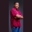Related Tags

swift
left shift
bitwise
communitycreator

# How to perform the bitwise right-shift operation in SwiftTheodore Kelechukwu Onyejiaku

### Overview

In Swift, we perform a bitwise left-shift operation using the operator <<. This operator requires two operands:

• The value we want to operate on.
• The number of times n to move the bits' position.

Just as the name implies, it first converts the number to binary and shifts the bits by the number of times specified.

For example, the bits that make up 2 are 0010 with a bitwise left-shift operation using 1 as the number of shifts. Then we'll have the result as 0100, which becomes 4.

### Syntax

value << n
Syntax for bitwise left-shift operator in Swift

### Parameters

value : This is the value we want to left-shift the bits of.

n: This is the number of times to left-shift the bits of the value.

### Return value

The value returned is a decimal value equivalent to the binary obtained after shifting the bits of value.

### Example

import Swift
// create some values
var v1 = 2;
var v2 = 5;
var v3 = 100;

// create some values representing the number of shifts
var n1 = 1;
var n2 = 4;
var n3 = 5;

// perform left shift operations
print(v1 << n1)  // 4
print(v2 << n2)  // 4
print(v3 << n3)  // 4
Left-shift the bits of a value in Swift

### Explanation

• Lines 3–5: We create some values (v1, v2, v3) which we want to left-shift the bits of.
• Lines 8–10: We create the number of shifts (n1, n2, n3) we want to perform in our bitwise left-shift operation.
• Lines 13–15: We perform the operation with the bitwise left-shift operator << and print the results to the console.

RELATED TAGS

swift
left shift
bitwise
communitycreator

CONTRIBUTORTheodore Kelechukwu Onyejiaku
RELATED COURSES

View all Courses

Keep Exploring

Learn in-demand tech skills in half the time